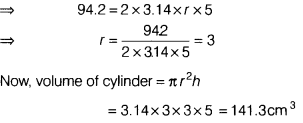# If the curved surface area of a cylinder is 94.2 cm2 and height is 5 cm

If the curved surface area of a cylinder is 94.2 cm2 and height is 5 cm, then find radius of its base and volume of the cylinder, [use π =3.14]Let radius of cylinder be r cm.
∴ Curved surface area of cylinder = 2πrh